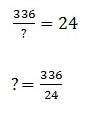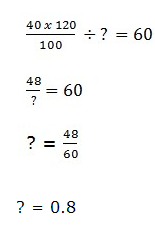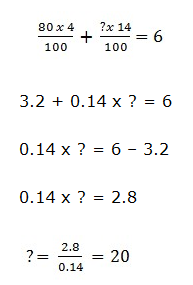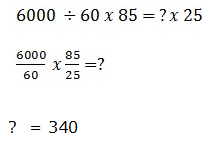# IBPS PO Prelims Quantitative Aptitude Questions 2021 (Day-06)

Dear Aspirants, Our IBPS Guide team is providing new series of Quantitative Aptitude Questions for IBPS PO Prelims 2021 so the aspirants can practice it on a daily basis. These questions are framed by our skilled experts after understanding your needs thoroughly. Aspirants can practice these new series questions daily to familiarize with the exact exam pattern and make your preparation effective.

Start Quiz

Wrong number series

Directions (01-05): Find out the wrong number in the following number series.

1) 2.5, 5, 25, 200, 2201, 30800

A.2.5

B.200

C.2201

D.25

E.5

2) 70, 68, 135, 395, 1575, 7869

A.1575

B.68

C.135

D.70

E.5395

3) 643, 768, 805, 1147, 1211, 1940

A.805

B.643

C.768

D.1211

E.1940

4) 8, 5, 6, 11, 21, 53.5

A.53.5

B.11

C.21

D.5

E.6

5) 339, 341, 345, 353, 369, 400

A.400

B.341

C.345

D.353

E.339

Simplification

Directions (06-09): What will come in place of question mark in the following questions?

6) 336 ÷ ? – 18 = 6

A.12

B.10

C.15

D.16

E.14

7) 40% of 120 ÷ ? = 60

A.0.8

B.1

C.1.2

D.1.4

E.0.6

8) 159 + √? + 21 = 142

A.296

B.225

C.144

D.216

E.256

9) 80 % of 4 + ? % of 14 = 6

A.16

B.20

C.25

D.14

E.18

Approximation

Directions (10): What approximate value should come in place of question mark in the following questions?

10) 5999.58 ÷ 60.005 × 85.232 = ? × 24.96

A.340

B.360

C.400

D.300

E.280

### Try IBPS PO Prelims Free Online Mock Test

2.5 x 2 = 5

5 x 5 = 25

25 x 8 = 200

200 x 11 = 2200

2200 x 14 = 30800

70 x 1 – 2 =68

68 x 2 – 3 = 133

133 x 3 – 4 = 395

395 x 4 – 5 = 1575

1575 x 5 -6 = 7869

643 + 53 = 768

768 + 62 = 804

804 + 73 = 1147

1147 + 82 = 1211

1211 + 93 = 1940

8 x 0.5 +1 = 5

5 x 1 + 1 = 6

6 x 1.5 + 1 = 10

10 x 2 + 1 = 21

21 x 2.5 +1 = 53.5

339 + 21 = 341

341 + 22 = 345

345 + 23 = 353

353 + 24 = 369

369 + 25 = 401? = 14

40% of 120 ÷ ? = 60√? = 196 – 180

√? = 16

? = 256For More Days IBPS PO Prelims Practice Set 2021

Get Quantitative Aptitude Study Materials

Get All Section Study Materials